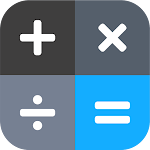ID: com.calculatorapp.simplecalculator.calculator

## The description of Basic Calculator: GPA & Math

Welcome to the Simple Calculator app! With this math calculator app, you can:
✔️ Currency conversion calculator
✔️ Unit conversion calculator
✔️ World time conversion calculator
✔️ Health Calculator
✔️ GPA calculator
✔️ Pro calculator math

This phone calculator plus app allows you to easily handle all the calculations necessary for everyday life. You can add or remove the functions and constants you need, calculate anything. With all the scientific calculations capability, this easy calculator app is the perfect choice for work and school.

The main feature of basic calculator app
1. General calculator utilities
• Supports 4 basic arithmetic: operations, squaring, parentheses of expressions, and simple scientific operations like: trigonometric and logarithmic functions.
• Perfect calculator math solver, calculator for all math problems
• Quick and easy calculation.
• Modify mistyped expressions with a freely moving cursor.
• Calculation history available

2. Unit conversion calculator app
• Supports length, weight, width, volume, time, temperature, pressure, speed, fuel efficiency and amount of data.
• Unit conversion app offline
• Supports all unit conversion calculations commonly used in daily life.

3. Currency conversion calculator
• Support calculator to convert 135 world currencies, including dollar, euro, yen, yuan, etc.
• Automatically calculates using real-time exchange rate.

4. World time conversion calculator
• Time conversion calculator for 400 or more world cities.
• Daylight savings time will also be reflected in this calculation.

5. Health calculator app
• You can measure your body mass index (BMI) and basal metabolic rate (BMR) with this simple calculator tool

6. GPA calculator app
• You can correctly calculate your GPA!

General calculator app is an easy basic calculator app for daily simple use, the basic calculator that you need for unit converter and calculations. The calculator pro app offers all the regular and scientific calculator functions. This calculator math app sums up all the calculations you need in life, making your life easier than ever.

👉 Hope this smart calculator application will help you in life, make your life faster and easier. This business math calculator app is still in development so your feedback is welcome. If you have any questions about math calculator app, don't hesitate to contact support email: [email protected]

Have a nice day 💕